# Congruence of Triangles – Everyday Mathematics

Example 1. Anaya is designing the window shown in the figure. She wants to make $$\Delta PRQ$$ congruent to  $$\Delta PRS$$. She designs the window so that  $$PR\bot QS$$. Which of the following conditions will make the two triangles congruent?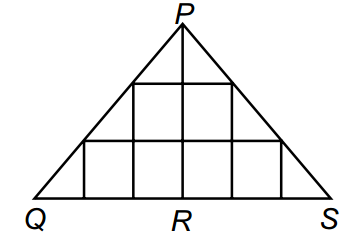A. RQ = RS

B. PQ = PS

C. Both (A) and (B)

D. None of these

Solution:

C

Example 2. Two satellites are being lauched such that their distance while moving in their respective orbits, are equal from Earth and Moon.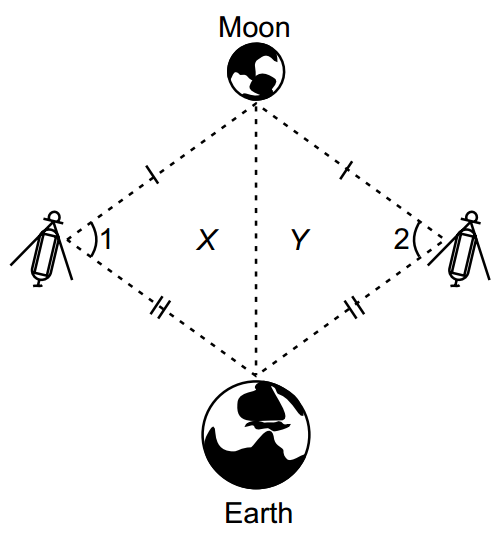Which of the following statements is/are true?

A. Figure X is not congruent to figure Y.

B. $$\angle 1$$ and  $$\angle 2$$ are not equal.

C. Both (A) and (B)

D. None of these.

Solution: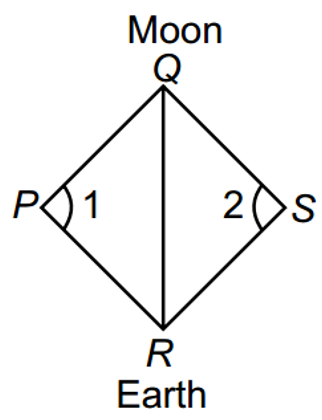D:

In  $$\Delta PQR$$ and  $$\Delta SQR$$

PQ = SQ (given)

PR = SR (given)

QR = QR (common)

$$\therefore \text{ }\Delta PQR\cong \Delta SQR$$ (By SSS)

$$\Rightarrow \angle 1=\angle 2$$ (By CPCT)

Example 3. Akira gave a problem to her sister Kiara. However, Kiara got stuck. Help Kiara identify whether the triangles are congruent and choose the correct option.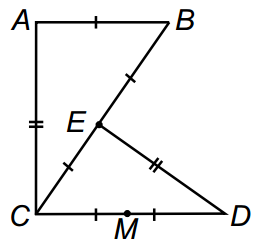A. Yes, $$\Delta ABC\cong \Delta DCE$$

B. No, they are not congruent

C. Yes, $$\Delta DCE\cong \Delta CAB$$

D. Yes,  $$\Delta DEC\cong \Delta CAB$$

Solution:

D: In the given figure,

MD = MC = EC – EB = AB

$$\Rightarrow DC=MD+MC=CE+EB=CB$$     (1)

Also, AB = EC   (2)

In  $$\Delta DEC$$ and  $$\Delta CAB$$

DE = CA (given)

DC = CB (from (1))

EC = AB (from (2))

$$\therefore \text{ }\Delta DEC\cong \Delta CAB$$  (By SSS)

Example 4. Three students Pia, Sia and Tia wrote a statement on a blackboard.

Pia wrote, “All rectangles are congruent”.

Sia wrote, “All equilateral triangles are congruent”.

Tia wrote, “All right angled triangles are congruent”.

Who wrote the INCORRECT statement?

A. Pia

B. Sia

C. Tia

D. All of them

Solution:

D

Example 5. Tiara wants to know the width of the given river. While doing so, she stands on the edge of the river and look staight across to a point on the other edge without changing the inclination of the neck and head. She turns side ways until the vision is in line with a point on the side of the steam.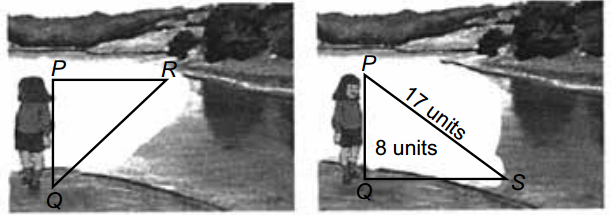A. 25 units

B. 12 units

C. 15 units

D. Can’t be determined

Solution:

C: In  $$\Delta PQS$$,

$$P{{S}^{2}}=P{{Q}^{2}}+Q{{S}^{2}}\Rightarrow {{17}^{2}}={{8}^{2}}+Q{{S}^{2}}$$

$$\Rightarrow Q{{S}^{2}}=289-64=225\Rightarrow QS=15$$.

From given conditions, RQ = QS (By CPCT) ( $$\because \text{ }\Delta PQR\cong \Delta PQS$$)

$$\therefore QR=15\text{ }units$$.